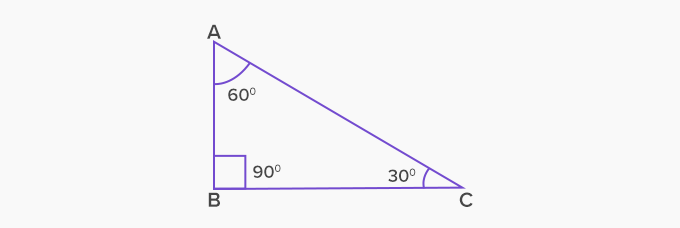# Right Triangle - Definition with Examples

The Complete K-5 Math Learning Program Built for Your Child

• 40 Million Kids

Loved by kids and parent worldwide

• 50,000 Schools

Trusted by teachers across schools

• Comprehensive Curriculum

Aligned to Common Core

What is Right Triangle?

A triangle in which one of the interior angles is 90° is called a right triangle. The longest side of the right triangle, which is also the side opposite the right angle, is the hypotenuse and the two arms of the right angle are the height and the base. Here’s what a right triangle looks like:Types of right triangles

Broadly, right triangles can be categorized as:

1. Isosceles right triangle: In this triangle, one interior angle measures 90° , and the other two angles measure 45° each. It is also known as a 45-90-45 triangle.This is an isosceles right triangle, with ∠A and ∠C measuring 45° each, and ∠B measuring 90° . Here, the sides AB and AC are equal because of the property that states that sides opposite to equal angles are also equal.

2. Scalene right triangle: This triangle is the one in which one interior angle measures 90°, while the other two measure 30° and 60°.In the scalene right triangle ABC, ∠A measures 30°, ∠B measures 90° and ∠C measures 60°. In this triangle, all the three sides will be of different lengths, and the three angles will be of different measures.

Right triangles around us:

Right triangles play a significant role in the field of architecture, astronomy, navigation, and surveying. Here are a few examples of right triangles that can be seen in the surroundings:Fun facts about right triangles: In the present scenario, right triangles play an important role in the field of architecture. The concept of right triangles was used by Egyptians to design pyramids.

Won Numerous Awards & Honors